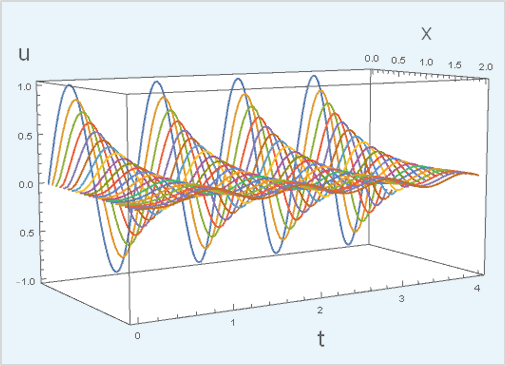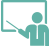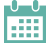#Wolfram U

Instructor-Led Course

# The Wolfram Language: Solving ODEs and DAEs with NDSolve

2hr
Intermediate
1 Certification
Estimated Time: 2hr
Course Level: Intermediate
Requirements: This course requires basic working knowledge of the Wolfram Language and of differential equations.
Certification Levels: Course completionThis course covers techniques for solving ordinary differential equations (ODEs) and differential-algebraic equations (DAEs) using the Wolfram Language. It introduces the built-in Wolfram Language function DSolve for finding symbolic solutions to differential equations and the built-in function NDSolve, a general numerical differential equation solver. Related functions and the options associated with them are demonstrated with examples.

Featured Products & Technologies: Wolfram Language, Mathematica

DateTimeLanguageLocationCost
This course is not currently scheduled.This course is available for custom training. »View all scheduled courses and events. »

### Outline

• Basics of using NDSolve
• Specifying boundary conditions
• Using options to control the numerical accuracy of the solution
• Selecting numerical methods to solve ODEs
• Working with stiffness in differential equations
• Including events in solutions of differential equations
• Solving boundary value and eigenvalue problems
• Properties of DAEs
• Applying index reduction, simplification and initialization methods